• ## MATLAB实现多元非线性回归

万次阅读 多人点赞 2019-01-10 16:25:36
简单多元非线性回归算例 现有以下数据 i x1 x2 x3 y 1 1.1 2 3.2 10.1 2 1 2 3.2 10.2 3 1.2 1.8 3 10 4 1.1 1.9 2.9 10.1 5 0.9 2.1 2.9 10 假如有以下模型： y=ax1+bx2+cx32y=ax_1+bx_2+cx_3^2y...
简单多元非线性回归算例
现有以下数据

i
x1
x2
x3
y

1
1.1
2
3.2
10.1

2
1
2
3.2
10.2

3
1.2
1.8
3
10

4
1.1
1.9
2.9
10.1

5
0.9
2.1
2.9
10

假如有以下模型：
$y=ax_1+bx_2+cx_3^2$
可以基于最小二乘法原理建立如下法方程组，其解则是上述系数.
$\sum_{j=0}^{n}(φ_j,φ_k ) a_j=(f,φ_k ),k=0,1,…n$
其中$n=2, φ_0=x1，φ_1=x2，φ_2=x3,f=y$.
MATLAB代码如下
x1=[1.1 1 1.2 1.1 0.9]';
x2=[2 2 1.8 1.9 2.1]';
x3=[3.2 3.2 3 2.9 2.9]';
y=[10.1 10.2 10 10.1 10]';
G=[x1,x2,x3.^2];
z=G\y;

得到$z=(3.296, 3.4404, -0.0141)^T$
所以有所以有
$y=3.296x_1+3.4404x_2-0.0141x_3^2$
或者可以调用MATLAB中的nlinfit函数。
[beta,r]=nlinfit(X,y,myfun,beta0);

其中输入参数X是自变量，y是因变量，myfun 是函数模型，beta0是初始迭代系数向量，返回值beta是模型的系数向量，r是每个点的残差。
先定义myfun
function y=myfun(beta,x)
y=beta(1)*x(:,1)+beta(2)*x(:,2)+beta(3)*x(:,3).^2;

然后主程序
x1=[1.1 1 1.2 1.1 0.9]';
x2=[2 2 1.8 1.9 2.1]';
x3=[3.2 3.2 3 2.9 2.9]';
y=[10.1 10.2 10 10.1 10]';
X=[x1,x2,x3];
beta0=[1,1,1];
[beta,r]=nlinfit(X,y,'myfun',beta0);

得到$beta=(3.296, 3.4404, -0.0141)^T$
所以有
$y=3.296x_1+3.4404x_2-0.0141x_3^2$
这边只涉及到如何获得回归方程中的系数，至于回归方程的优劣性判断，暂不做讨论。


展开全文matlab
• 对实验获得的离散点进行拟合,采用最小二乘原理多元非线性回归模型线性化理论计算并确定模型参数,建立半焦分选特性的多元非线性回归模型,模型计算结果与实验数据吻合较好,相对误差均在9%以内。
介绍两种方法做多元非线性回归：lsqcurvefit、Adagrad 法。

clear; clc;
% 多元非线性回归模型
% z = w1 * exp(-x / w2) + w3 * y
% z = 10 * exp(-x / 5) + 2 * y
len = 20;
rng('default');
x = randi(len + 1, len, 1) / 5;
y = randi(len + 1, len, 1) / 5;
z = 10 * exp(-x / 2) + 0.5 * y;
ratio = 0.0;
z = z + ratio * max(z) * rand(len, 1);
X = [x, y];
fun = @(var, X)var(1) * exp(-X(:, 1) / var(2)) + var(3) * X(:, 2);
w = lsqcurvefit(fun, [1, 1, 1], X, z);
disp(['lsqcurvefit 计算结果：', num2str(w)]);
% 梯度下降法学习
% obj = 1 / (2 * len) * (w1 * exp(-x / w2) + w3 * y - z)' * (w1 * exp(-x / w2) + w3 * y - z)
alpha = 5;   % 学习率大收敛快，可能有震荡
iteMax = 10000;
w1 = 1;
w2 = 1;
w3 = 1;
initW = [w1; w2; w3];
err = 1e-6;
J = zeros(iteMax, 1);
G = zeros(3, 1);
e = 0.1;
for i = 1 : iteMax
gradW1 = 1 / len * (exp(-x / w2))' * (w1 * exp(-x / w2) + w3 * y - z);
gradW2 = 1 / len * (w1 * x .* exp(-x / w2) / w2^2)' * (w1 * exp(-x / w2) + w3 * y - z);
gradW3 = 1 / len * y' * (w1 * exp(-x / w2) + w3 * y - z);
newW = initW - alpha * diag(1 ./ sqrt(G + e)) * grad;
if norm(newW - initW) < err
J(i + 1 : end) = [];
disp(['梯度下降法迭代次数：', num2str(i)]);
disp(['梯度下降法迭代结果：', num2str(newW')]);
break;
else
initW = newW;
w1 = newW(1);
w2 = newW(2);
w3 = newW(3);
J(i) = 1 / (2 * len) * (w1 * exp(-x / w2) + w3 * y - z)' * (w1 * exp(-x / w2) + w3 * y - z);
end
end
% 绘图
subplot(1, 2, 1)
loglog(J, 'LineWidth', 2)
legend(['alpha = ', num2str(alpha)]);
xFit1 = linspace(min(x), max(x), 30);
yFit1 = linspace(min(y), max(y), 30);
[xFit2, yFit2] = meshgrid(xFit1, yFit1);
zFit = w1 * exp(-xFit2 / w2) + w3 * yFit2;
subplot(1, 2, 2)
scatter3(x, y, z, 'filled');
hold on
mesh(xFit2, yFit2, zFit);
hold off
legend('Points', 'Fitting', 'Location','NorthOutside');

结果打印


Local minimum found.

Optimization completed because the size of the gradient is less than
the default value of the function tolerance.

<stopping criteria details>

lsqcurvefit 计算结果：10           2         0.5
梯度下降法迭代次数：1934
梯度下降法迭代结果：10            2          0.5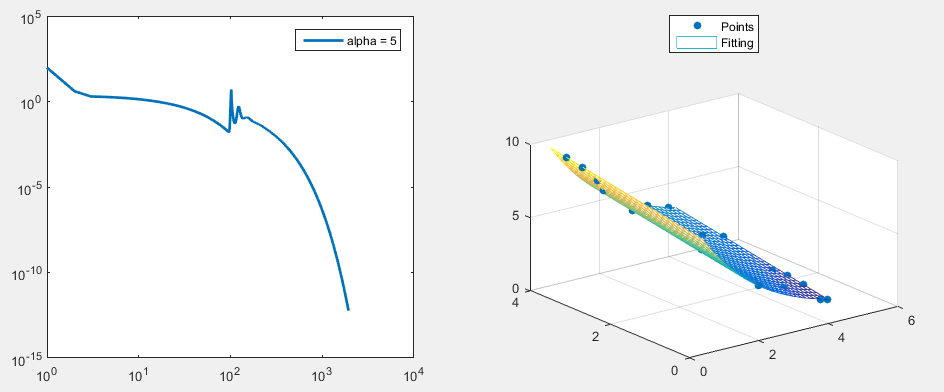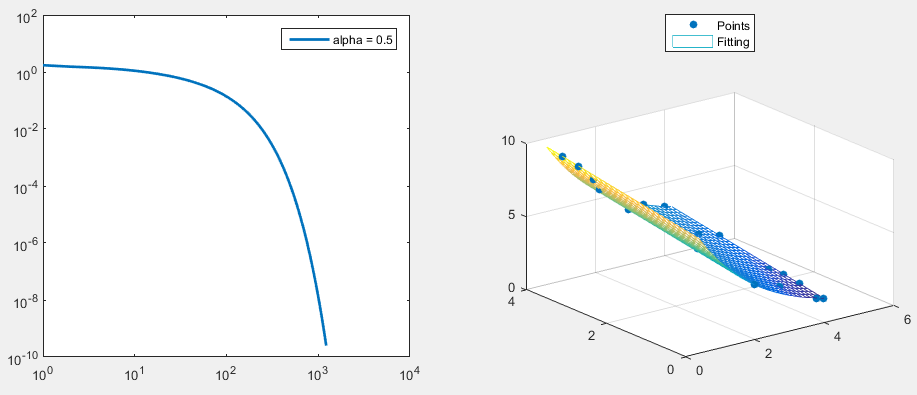展开全文• 在回归分析中，如果有两个或两个以上的自变量，就...看到regress函数的例子，突然想到它居然可以用来做一些简单的多元非线性回归分析，例如，我们用它对一个多项式进行拟合，将要拟合的多项式进行展开，得到的其实...
在回归分析中，如果有两个或两个以上的自变量，就称为多元回归。事实上，一种现象常常是与多个因素相联系的，由多个自变量的最优组合共同来预测或估计因变量，比只用一个自变量进行预测或估计更有效，更符合实际。因此多元线性回归比一元线性回归的实用意义更大。看到regress函数的例子，突然想到它居然可以用来做一些简单的多元非线性回归分析，例如，我们用它对一个多项式进行拟合，将要拟合的多项式进行展开，得到的其实就是一个类似因变量y与多个自变量x1, x2...xn之间的表达式：Y= β0+ β1X1+β2X2+……+βpXp+e。clearclcdata = [55555551010101010101015151515151515202020202020202525252525252530303030303030144176208240272320512144176208240272320512144176208240272320512144176208240272320512144176208240272320512144176208240272320512135.346138.193147.369137.871140.782143.022146.985179.075230.787236.99240.18232.734248.473249.812218.834242.56276.038282.54293.582320.17279.204215.68286.505313.645334.782325.526332.145337.282231.54261.884324.214343.436367.167372.446340.181259.584302.132313.351347.63340.478414.03443.249]';x1 = data(:,1);x2 = data(:,2);y = data(:,3);X = [ones(size(x1)) x1.*x1 x1 x2.*x2 x2 x1.*x2];[b,bint] = regress(y,X)scatter3(x1,x2,y,'filled')hold onx1fit = min(x1):0.5:max(x1);x2fit = min(x2):0.5:max(x2);[X1FIT,X2FIT] = meshgrid(x1fit,x2fit);YFIT = b(1)+ b(2)*X1FIT.*X1FIT+b(3)*X1FIT + b(4)*X2FIT.*X2FIT + b(5)*X2FIT + b(6)*X1FIT.*X2FIT;mesh(X1FIT,X2FIT,YFIT)xlabel('x1')ylabel('x2')zlabel('Y')view(140,30)result:b =-62.4591-0.310514.1703-0.00160.96650.0159bint =-127.4290    2.5108-0.4143   -0.206710.0531   18.2875-0.0021   -0.00100.6079    1.32500.0092    0.0226当然，你也可以自定义一个这样的函数y = (a*x1+b*x2)^2，用非线性拟合函数nlinfit进行验证。
展开全文• ## 多元线性回归原理

千次阅读 2019-03-27 21:36:17
例子先行 一元线性：拿房价预测来说，房价就只与一个特征size（feet^2）有关: ... age of home....,为了引入这些特征，有了多元线性回归，x1,x2,x3,x4为特征，y为预测的价格。 符号引入： n：特征的数量 ...
例子先行

一元线性：拿房价预测来说，房价就只与一个特征size（feet^2）有关: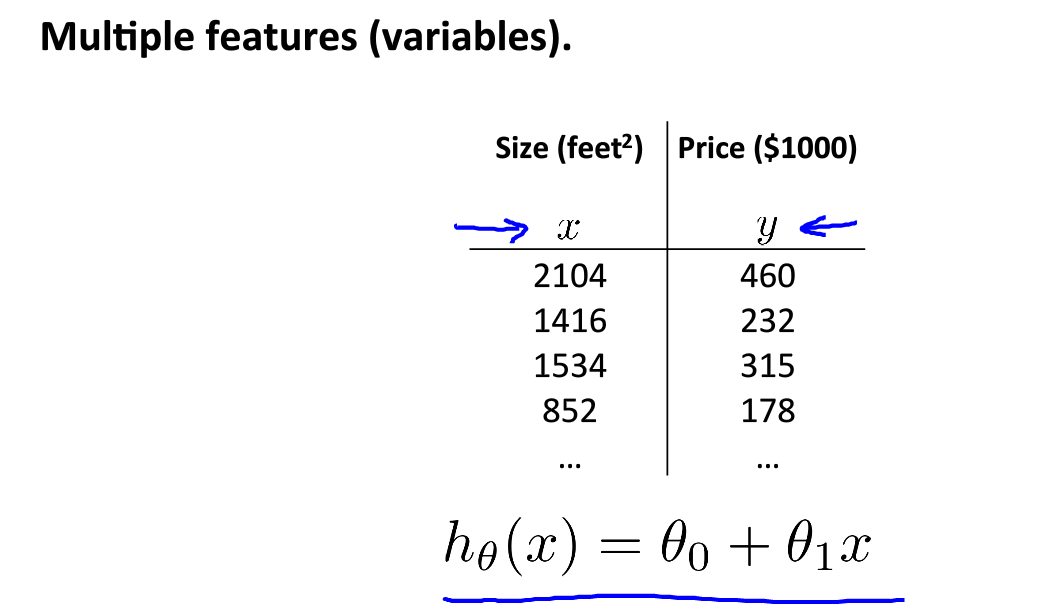多元线性：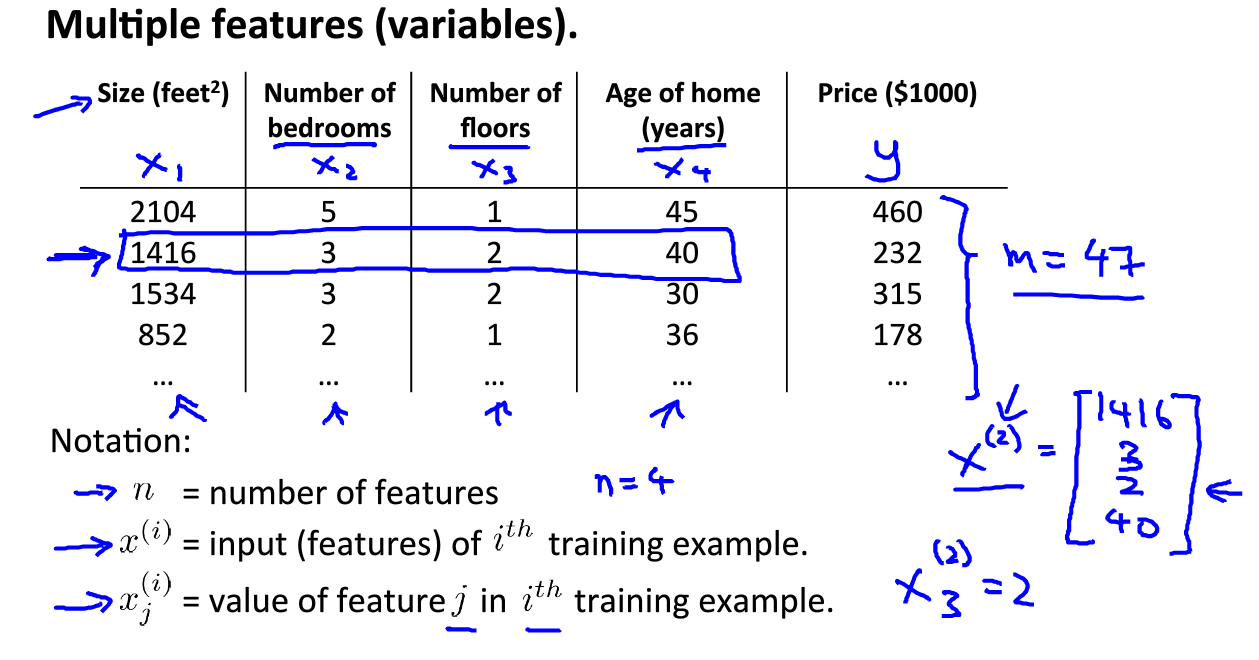房子除了面积，还会有其他的特征：number of bedrooms;number of floors; age of home....,为了引入这些特征，有了多元线性回归，x1,x2,x3,x4为特征，y为预测的价格。

符号引入：

n：特征的数量

:第i个训练样例

:第i个训练样例的第j个特征

可以得到模型函数：

我们要假设x0为1，即（）,可以理解为我们增加了一个额外的第零个特征向量，因此我们的特征向量由原来的n个变为了n+1个，所以现在我们X的特征向量为：,n+1维向量，的n+1维向量，则向量表示模型函数为：

,   而，所以内积

定义代价函数：

如图将模型写成向量形式，用向量代替，代价函数用向量J()代替，梯度下降的代价函数也用向量J()代替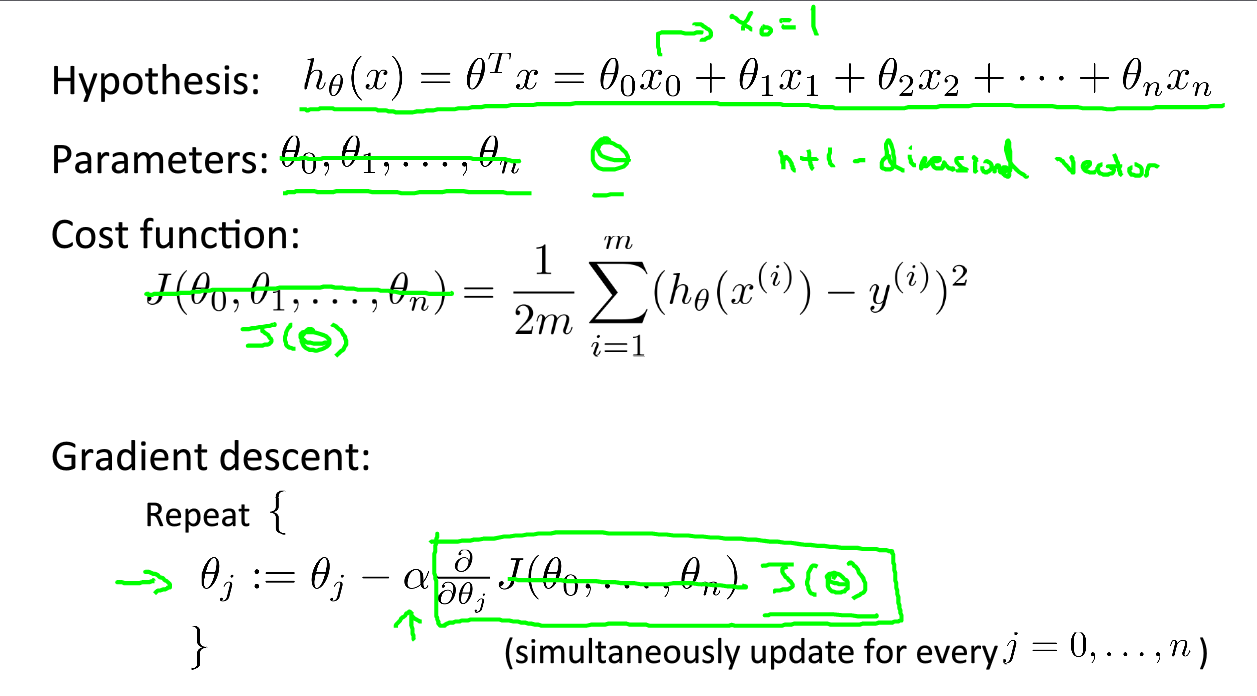从0～n不断的update第j个特征的参数，即执行梯度下降的过程。下图是一元和多元的比较，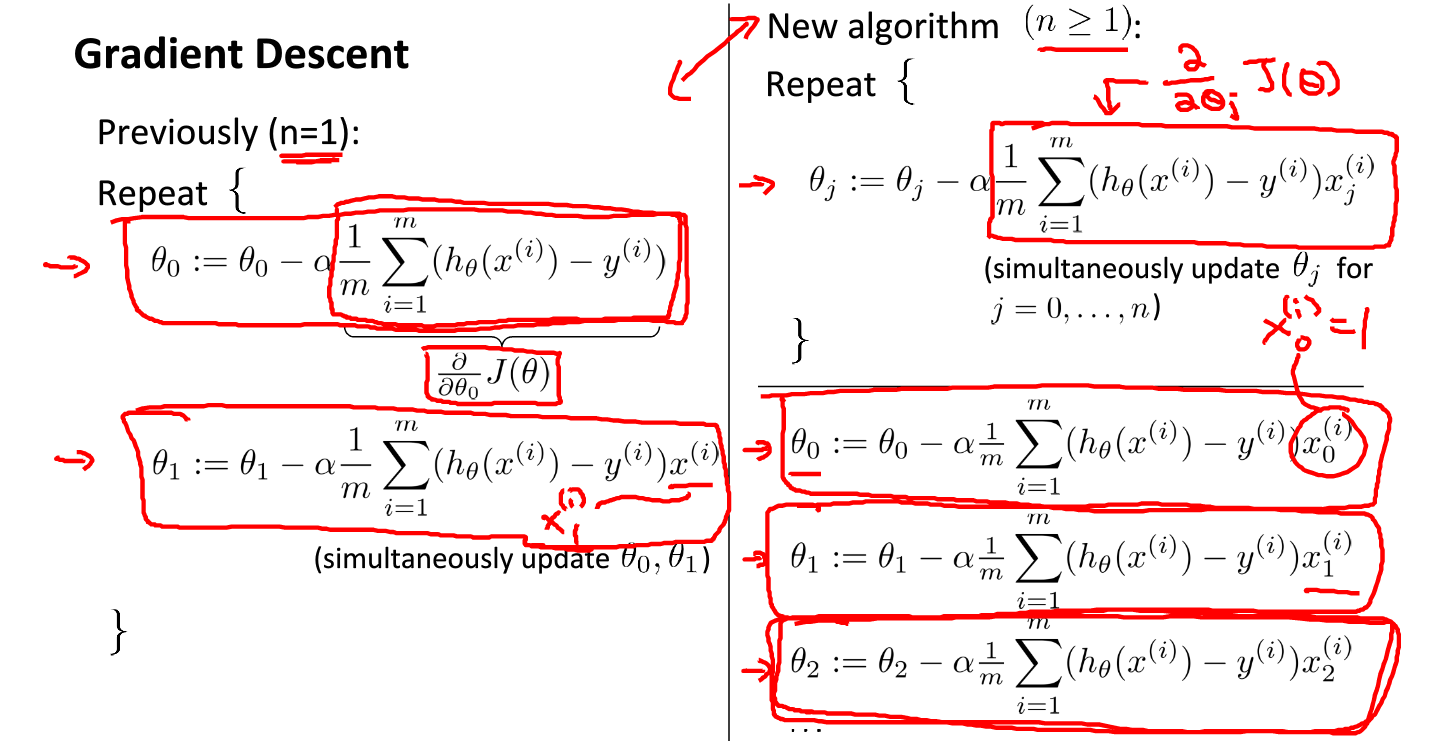具体求导过程和一元的类似，如下是推理证明过程。

多元线性回归思路

经过简单的一元线性回归知识，可以了解知道，多元线性回归是将原来一元的x变成了x的向量，由y=b+a*x到了y=a0+a1*X1+a2*X2+...+an*Xn,含有多个特征。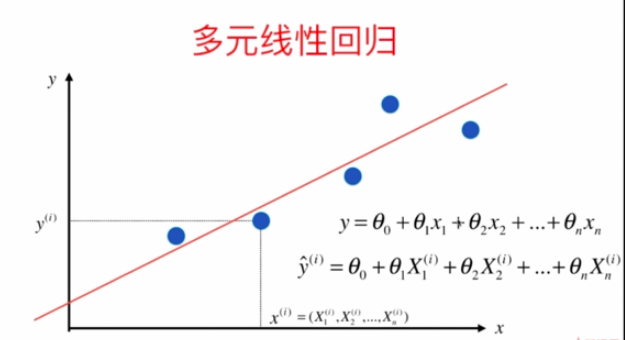得到一个直线（实际不是直线，有可能是面或者跟复杂的曲线（面））最大程度的拟合所有的点，

方程：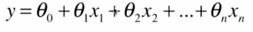，x向量（x1~xn）和y都是已知的，只需要求出即可，所求出来的也是一个向量，即求得了线性回归的模型。

先列举几个几个典型的模型：

● 最基本的单变量线性回归：
形如h(x)=theta0+theta1*x1
● 多变量线性回归：
形如h(x)=theta0+theta1*x1+theta2*x2+theta3*x3
● 多项式回归（Polynomial Regression）：
形如h(x)=theta0+theta1*x1+theta2*(x2^2)+theta3*(x3^3)
或者h(x)=ttheta0+theta1*x1+theta2*sqr(x2)
但是可以令x2=x2^2，x3=x3^3，于是又将其转化为了线性回归模型。虽然不能说多项式回归问题属于线性回归问题，但是一般我们就是这么做的。
● 所以最终通用表达式就是：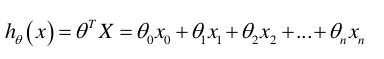原理思路：

由最小二乘法，多元线性回归的损失函数：和一元线性回归一样都是求的最小值，让预测结果和真实的结果的差值的平方 尽可能的小。

是个向量，x也是个向量，原来为列向量，让变为行向量，转置

对做一个改变，在前面也乘以一个系数,为了不改变原式子，令=1.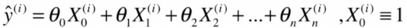所以,由此可以变成向量形式：=

推广到整个式子为：,其中和分别为（m,n+1）的矩阵和（n+1,1）的矩阵，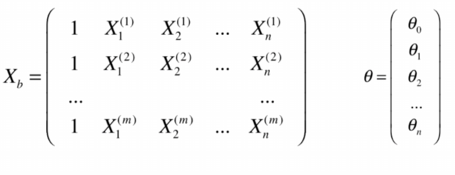所得结果为 所有的预测值组成的向量 。根据矩阵乘法法则，最后得到的是一个（m，1）的矩阵。

我们的目标就是让代价函数最小：最小，变为向量形式为：，将带入上式子得：

,在目标函数中，对第一个 进行了转置，这是为了方便使用矩阵的乘法；转置之后，第一个式子为（1，m）的行向量，第二个式子为（m,1）的列向量；所以最终结果为一个值。

最小二乘法求多元线性回归的参数

在学习模型的任务中，我们要做到的是让预测值尽量逼近真实值，做到误差最小，而均方误差就是表达这种误差的一种，所以我们要求解多元线性回归模型，就是要求解使均方误差最小化时所对应的参数：(其中W 相当于)式（1）

其中w*为模型对应的解，即使得均方误差函数最小化时的权重向量。

那么，我们应该如何求w*呢？在这里，我们可以用最小二乘法对模型的参数进行估计，具体做法是：损失函数对需要求解的参数进行求导（求梯度），并且令其导数为0，求得相应的参数。

在这里，我们需要让对w求导，在求导之前，我们来看一下两个求导公式：式（2）式（3）

下图为详细的求导过程:损失函数对参数进行求导之后，可以求得：式（4）

令式（4）为零可得:式（5）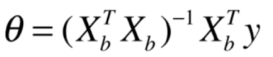以上即为参数w最优解的闭式解，但我们可以发现w*的计算涉及矩阵的求逆，这样的话就有一些限制了，只有在X^T*X为满秩矩阵或者正定矩阵时，才可以使用以上式子计算。但在现实任务中，X^T*X往往不是满秩矩阵，这样的话就会导致有多个解，并且这多个解都能使均方误差最小化，但并不是所有的解都适合于做预测任务，因为某些解可能会产生过拟合的问题。

缺点：时间复杂度高：O(n^3) (优化O(n^2.4))； 当我们有上百万个样本，或者上百万个特征的时候，运算时间会非常长；但是能直接求得数学解也是很不错的，因为在机器学习算法中，很少有算法可以直接求出数学解。

优点：不需要对数据做归一化处理；结果是 原始数据运算得到结果，所以不存在量纲的问题；

参考：https://www.cnblogs.com/pengyingzhi/p/5383801.html

https://blog.csdn.net/qq_37610062/article/details/82532995

展开全文• ## 多元线性回归方程原理及其推导

万次阅读 多人点赞 2018-10-21 14:19:22
多元线性方程原理及推导 概念 1.在统计学中，线性回归方程是利用最小二乘函数对一个或多个自变量之间关系进行建模的一种回归分析。这种函数是一个或多个称为回归系数的模型参数的线性组合。只有一个自变量的情况...
• 线性回归1.1 基本形式1.2 最小二乘法推导2. Sklearn 实现参考资料 相关文章：机器学习 | 回归评估指标 1. 线性回归 线性回归，又称普通最小二乘法（Ordinary Least Squares, OLS），是回归问题最简单也最经典的...机器学习 监督学习 Python Sklearn
• 请在页面菜单里查找。...其他章节都以这个定义为基础，后面每一章里介绍的机器学习模型都是按照这个思路解决...介绍线性回归模型，一种解释变量和模型参数与连续的响应变量相关的模型。本章介绍成本函数的定义，通过最...
• 在回归分析中，如果有两个或两个以上的自变量，就称为多元回归。...因此多元线性回归比一元线性回归的实用意义更大。  很久没有用MATLAB了，今天在http://www.mathworks.com/help/toolbox/stats/regress.htmlmatlab
• 监督学习 | 线性回归 之多元线性回归原理及Sklearn实现 监督学习 | 线性回归 之正则线性模型原理及Sklearn实现 1. 多项式回归 对于非线性数据，也可以用线性模型来拟合。一个简单的方法就是将每个特征的幂次方...机器学习 监督学习 Sklearn Python
• 二、多元线性回归 1、原理 2、最小二乘法求解多元线性回归的参数 3、sklearn练习 三、回归类模型评估指标 1、是否预测到了正确的数值 2、是否拟合到了足够的信息 四、岭回归和Lasso 1、多重共线性 2、岭回归 3、...
• 本课程主要讲述如何使用python进行线性回归与非线性回归分析，包括： 基于statsmodel的线性回归方法 基于sklearn的线性回归方法 基于Numpy的一元多项式非线性回归方法 基于sklearn的多元多项式非线性回归方法 基于...大数据 大数据技术 大数据平台 大数据学习路线
• 线性回归非线性回归TŤLinear Regression is famously known for being a simple algorithm and a good baseline to compare more complex models to. In this article, we explore the algorithm, understand the ...python 机器学习
• 二、实现多元线性回归**数据标准化****特征值排序，检验是否为病态回归问题****若为病态回归问题，则降维处理****建立回归模型，计算回归参数、回归结果****平方和分解****显著性检验****回归直线方程****求解置信...机器学习 统计学
• 前言 本文将简单介绍线性回归算法的原理以及推导过程，因为本人也是新手，只按照...否则是非线性回归，常见的非线性回归包括多项式回归、逻辑回归。通过样本学习映射关系f:x->y，得到的预测结果y是连续值变量。 ...机器学习 过拟合
• 多元线性回归算法和正规方程解 ——燕江依/2019.08.05 1、对于多元线性回归...2、对于KNN算法和多元线性回归算法对比可以知道，KNN算法是一种参数学习的算法，而多元线性回归算法是一种参数学习的算法，另外KN...
• 非线性回归 从这一步开始进入了两层神经网络的学习，从而解决非线性问题。 在两层神经网络之间，必须有激活函数连接，从而加入非线性因素，提高神经网络的能力。所以，我们先从激活函数学起，一类是挤压型的激活函数...深度学习 神经网络
• 与一元线性回归一样，多元线性回归自然是一个回归问题。只不过一元线性回归的方程可以写成。 y=ax+b 多元线性回归是 y=a1x1+a2X2+a3X3+…+anXn+b 相当于我们高中学的一元一次方程，变成了n元一次方程。因为y还是...
• 主要介绍了Python利用神经网络解决非线性回归问题,结合实例形式详细分析了Python使用神经网络解决非线性回归问题的相关原理与实现技巧,需要的朋友可以参考下
• 文章目录机器学习分类线性回归原理线性回归定义 机器学习分类 机器学习的主要任务便是聚焦于两个问题：分类和回归 分类相信大家都不会陌生，生活中会见到很多的应用，比如垃圾邮件识别、信用卡发放等等，...
• ## 线性回归原理分析

千次阅读 2019-04-20 23:07:24
线性回归原理详解回归问题定义问题求解欢迎使用Markdown编辑器新的改变功能快捷键合理的创建标题，有助于目录的生成如何改变文本的样式插入链接与图片如何插入一段漂亮的代码片生成一个适合你的列表创建一个表格设定...机器学习
• ## 多元线性回归的基础理解

万次阅读 多人点赞 2018-11-19 01:09:28
多元线性回归  Multivarate Linear Regression Multiple Feature 前面我们学习了一元线性回归，也动手亲自从底层编写了梯度下降算法来实现一元线性回归。相信大家已经对梯度下降和线性回归有了很清晰的理解了。 ...机器学习 梯度下降 正规方程 特征缩放
• 什么是线性回归线性回归是利用数理统计中的回归分析，来确定两种或两种以上变量间相互依赖的定量关系的一种统计分析方法，运用十分广泛。其表达形式为y = w'x+e，e为误差服从均值为0的正态分布。 简单来说：...大数据
• 回归一直是个很重要的主题...回归主要包括线性回归，非线性回归以及分类回归。本文主要讨论多元线性回归（包括一般多元回归，含有虚拟变量的多元回归，以及一点广义差分的知识）。请大家不要觉得本人偷奸耍滑，居然只有
• 文章目录原理以及公式【1】一元线性回归问题【2】多元线性回归问题【3】学习率【4】流程分析（一元线性回归）【5】流程分析（多元线性回归）归一化原理以及每种归一化适用的场合一元线性回归代码以及可视化结果多元...可视化 python 深度学习 机器学习 数据分析...